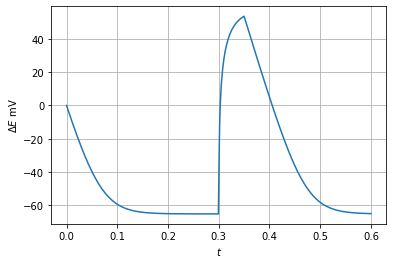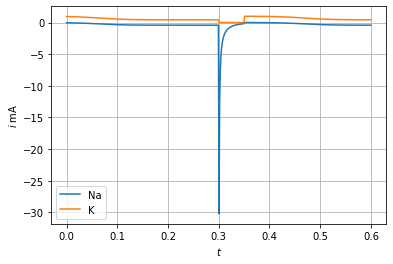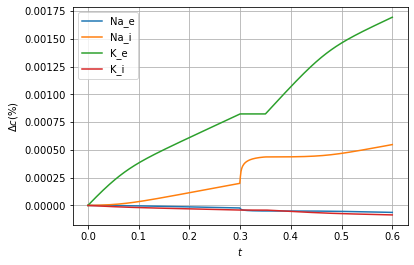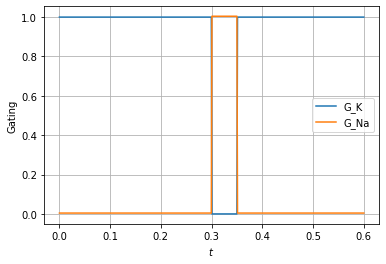Note:

• This example is discussed in detail by Gawthrop and Pan (2020) available here.

• This is the ElectroChemical.ipynb notebook. The PDF version is available here.

# Introduction¶

## Faraday equivalent potential¶

The bond graph approach uses the notion of energy covariables: a pair of variables whose product is power. Thus, for example, electrical systems have voltage (with units V) and current (with units A) as covariables and the product has units of power (W or Js$^{-1}$). Chemical system covariables are chemical potential $\mu$ (with units JC$^{-1}$) and molar flow $f$ (with units mol.s$^{-1}$)\citep{OstPerKat71,OstPerKat73,GawCra14}; again the product has units of power (W or Js$^{-1}$).

The commonality of power over different physical domains makes the bond graph approach particularly appropriate to model multi-domain systems, in particular chemoelectrical systems \citep{GawSieKam17}. Noting that the conversion factor relating the electrical and chemical domains is Faraday's constant $F\approx 96485{C.mol^{-1}}$. As discussed by \citet{Kar90} and \citet{GawSieKam17}, this conversion can be represented by the bond graph transformer (TF) component. An alternative approach introduced by \citet{Gaw17a} is to divide the covariables $\mu$ and $f$ by $F$ to give the pair of covariables $\phi$ and $f$ where: \begin{align} &\text{Faraday-equivalent chemical potential}& \phi &= \frac{\mu}{F} \text{V} \label{eq:phi}\\ &\text{Faraday-equivalent flow}& f &= F v \text{A} \label{eq:f} \end{align}

## Chemical properties¶

The Ce components representing chemical species generate Faraday-equivalent potential (FEP) $\phi$ (measured in Volts) in terms of the amount of species $x$ as: \begin{align} \phi &= \phi^\ominus + \phi_N \ln \frac{x}{x^\ominus}\\ &= \phi_N \ln K x\\ \text{where } K &= \frac{K^\ominus}{x^\ominus}\\ V_N &= \frac{RT}{F} \approx 26 mV\\ \text{and } K^\ominus &= \ln\frac{\phi^\ominus}{\phi_N} \end{align} $\phi^\ominus$ in the standard potential at the standard amount $x^\ominus$. $R$ is the universal gas constant and $F$ Faraday's constant.

The amount of species $x$ is the integral of the species flow $f$: \begin{equation} x = \int^t f(\tau)d\tau \end{equation}

The formula can also be expressed in terms of concentration $c$ as: \begin{align} \phi &= \phi_N \ln K_C^\prime c\\ \text{where } K_c^\prime &= \frac{K^\ominus}{c^\ominus}\\ \end{align} $c^\ominus$ is the concentration at standard conditions.

## Electrical properties¶

The C components representing electrical capacitance generate electrical potential $\phi$ (measured in Volts) in terms of the amount of positively charges $x$ and electrical capacitance $C$ as: \begin{align} \phi &= \frac{x}{C}\\ &= \phi_N K_E x_E\\ \text{where } K_E &= \frac{1}{x_N}\\ \text{and } x_N &= C\phi_N \end{align} The amount of charge $x_E$ is the integral of the charge flow (current) $f_E$: \begin{equation} x_E = \int^t f_E(\tau)d\tau \end{equation}

In :
## Some useful imports
import BondGraphTools as bgt
import numpy as np
import sympy as sp
import matplotlib.pyplot as plt

## Stoichiometric analysis
import stoich as st

## SVG
import svgBondGraph as sbg

## Display (eg disp.SVG(), disp.
import IPython.display as disp

quiet = True

## Fix the concentrations via chemostats
Fix_conc = False

/home/peterg/WEB/Github/Papers/GawPan20/stoich.py:38: SyntaxWarning: "is" with a literal. Did you mean "=="?
if name is 'x':
/home/peterg/WEB/Github/Papers/GawPan20/stoich.py:40: SyntaxWarning: "is" with a literal. Did you mean "=="?
elif name is 'u':
/home/peterg/WEB/Github/Papers/GawPan20/stoich.py:49: SyntaxWarning: "is" with a literal. Did you mean "=="?
if (name is 'u') and (comp.name is 'r'):
/home/peterg/WEB/Github/Papers/GawPan20/stoich.py:49: SyntaxWarning: "is" with a literal. Did you mean "=="?
if (name is 'u') and (comp.name is 'r'):
/home/peterg/WEB/Github/Papers/GawPan20/stoich.py:53: SyntaxWarning: "is" with a literal. Did you mean "=="?
if comp.metamodel is 'BG':
/home/peterg/WEB/Github/Papers/GawPan20/stoich.py:1469: SyntaxWarning: "is" with a literal. Did you mean "=="?
if invar is 'phi':

In :
## Concentrations in nM for Na and K in Giant Squid Axon
## From Keener & Sneyd Table 2.1
conc_e = {'Na':437, 'K':20}
conc_i = {'Na':50, 'K':397}


# Electrodiffusion¶

Cellular membranes have pores though which chemical species can diffuse. If the species are charged, the diffusion both depends on and creates an electrical potential. This section looks at a single ionic species with generic name I$^+$; this can be thought of as Na$^{+}$ or K$^{+}$.

The bond graph representation of a charged ion has three components: a Ce component to represent the chemical properties of the ion, a C component to repesent the electrical properties of the ion and a 1 junction to make the flow into the two components identical.

The resultant potential is then the sum of the chemical and electrical components: \begin{align} \phi &= \phi_C + \phi_E\\ \text{where } \phi_C &= \phi_N \ln K x\\ \text{and } \phi_E = \phi_N K_E x_E \end{align}

If the ion has two charges (I$^{++}$) the bold bonds in the diagram would each be replaced by two bonds; alternatively, if the ion had a negative charge (I$^{-}$) the bold bonds in the diagram would each be replaced by a bond with reversed direction.

The bond graph of the pore itself has two pools of charged ions: internal and external connected by a reaction (Re) component. As the ion in each pool is the same, the property $K^\prime$ is the same for each pool. Thus the reaction potential $\Phi$ is the difference of the potentials of the internal and external ion pools: \begin{equation} \Phi = \phi_N \left ( \ln K^\prime c_i - \ln K^\prime c_e \right )

• \left ( \phi{Ei} - \phi{Ee} \right ) \end{equation} Defining $\Delta E = \phi_{Ei} - \phi_{Ee}$ and noting that at equilibrium $\Phi=0$: \begin{equation} \Delta E = \phi_N \ln\frac{c_e}{c_i} \end{equation} This is the expresion for the Nernst potential for a species with a single positive charge.
In :
## Electrodiffusion
sbg.model('Electrodiffusion_abg.svg')
import Electrodiffusion_abg
disp.SVG('Electrodiffusion_abg.svg')

Out:
In :
## Stoichiometry: linear Re
s = st.stoich(Electrodiffusion_abg.model(),linear=['Ei','Ee','r'],quiet=quiet)

if Fix_conc:
chemostats = ['Ii','Ie']
else:
chemostats = []

sc = st.statify(s,chemostats=chemostats)

In :
## Stoichiometric matrix
disp.Latex(st.sprintl(s,'N'))

Out:
\begin{align} N &= \left(\begin{matrix}1\\-1\\1\\-1\end{matrix}\right) \end{align}
In :
## Reactions
disp.Latex(st.sprintrl(s,chemformula=True,all=True))

Out:
\begin{align} \ch{Ei + Ii &<>[ r ] Ee + Ie } \end{align}
In :
## Flows
disp.Latex(st.sprintvl(s))

Out:
\begin{align} v_{r} &= \kappa_{r} \left(- K_{Ee} x_{Ee} + K_{Ei} x_{Ei} - V_{N} \left(\log{\left(K_{Ie} x_{Ie} \right)} - \log{\left(K_{Ii} x_{Ii} \right)}\right)\right) \end{align}
In :
## Stoichiometry: nonlinear Re
s = st.stoich(Electrodiffusion_abg.model(),linear=['Ei','Ee'],quiet=quiet)

if Fix_conc:
chemostats = ['Ii','Ie']
else:
chemostats = []

sc = st.statify(s,chemostats=chemostats)

In :
## Reactions
disp.Latex(st.sprintrl(s,chemformula=True,all=True))

Out:
\begin{align} \ch{Ei + Ii &<>[ r ] Ee + Ie } \end{align}
In :
## Flows
print(st.sprintvl(s))
disp.Latex(st.sprintvl(s))

\begin{align}
v_{r} &= \kappa_{r} \left(- K_{Ie} x_{Ie} e^{\frac{K_{Ee} x_{Ee}}{V_{N}}} + K_{Ii} x_{Ii} e^{\frac{K_{Ei} x_{Ei}}{V_{N}}}\right)
\end{align}


Out:
\begin{align} v_{r} &= \kappa_{r} \left(- K_{Ie} x_{Ie} e^{\frac{K_{Ee} x_{Ee}}{V_{N}}} + K_{Ii} x_{Ii} e^{\frac{K_{Ei} x_{Ei}}{V_{N}}}\right) \end{align}
In :
## Set non-unit parameters
K_Ii = 1e-3
K_Ie = 1e-3
C = 1
def setPar(s,C=1,conc_i=1,conc_e=1,prefix=['']):
K_E = 1/C

## Parameters
parameter = {}
parameter['K_Ei'] = 0
parameter['K_Ee'] = K_E

## Initial state
sp = s['species']
re = s['reaction']
X0 = np.ones(s['n_X'])
X0[sp.index('Ei')] = 0
X0[sp.index('Ee')] = 0
for p in prefix:
## Parameters
KK = 'K_'+p
kk = 'kappa_'+p
parameter[KK+'Ii'] = K_Ii
parameter[KK+'Ie'] = K_Ie

## States and kappa
if len(p) == 0:
Ion = 'Na'
else:
Ion = p[0:len(p)-1]
#X0[sp.index('Ee')] = 0.077/K_E

print(Ion)
X0[sp.index(p+'Ii')] = conc_i[Ion]/K_Ii
X0[sp.index(p+'Ie')] = conc_e[Ion]/K_Ie
parameter[kk+'r'] = 1/conc_i[Ion]

return parameter,X0

In :
def CheckTheory(dat):

if 'Ii' in s['species']:
## Check Nernst potential
t = dat['t']
phi_Ei = dat['phi'][:,s['species'].index('Ei')]
phi_Ee = dat['phi'][:,s['species'].index('Ee')]
x_Ii = dat['X'][:,s['species'].index('Ii')]
x_Ie = dat['X'][:,s['species'].index('Ie')]
#     v = dat['V'][:,s['reaction'].index('r')]
V_N = st.V_N()

#     v_ss = v[-1]
dV = (phi_Ei[-1]-phi_Ee[-1] )
dV_theory = V_N*np.log(x_Ie[-1]/x_Ii[-1])
#     print(f'Steady-state flow is {v_ss:0.2}')
print(f'dV = {dV*1000:4.1f}mV')
print(f'dV_Theory = {dV_theory*1000:4.1f}mV')

In :
def Simulate(s,sc,T=1,X_chemo=None,prefix=['']):
## Time
t = np.linspace(0,T,500)

## Parameters and initial state
parameter,X0 = setPar(s,C=C,conc_i=conc_i,conc_e=conc_e,prefix=prefix)

## Simulate
dat = st.sim(s,sc=sc,t=t,parameter=parameter,X0=X0,X_chemo=X_chemo,quiet=True)

CheckTheory(dat)

return dat

In :
dat = Simulate(s,sc)
st.plot(s,dat,plotPhi=True,species=['Ei','Ee'])
st.plot(s,dat,plotPhi=True,species=['Ei','Ee']) ##,filename='Figs/electrodiffusion.pdf')

Na
dV = 57.9mV
dV_Theory = 57.9mV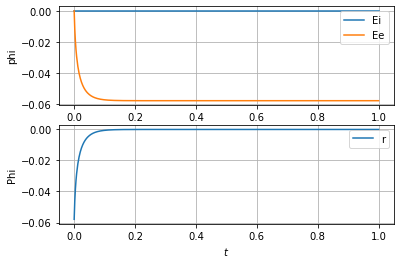## Voltage clamp¶

The voltage agross the membrane is clamped by setting C:Ei and C_Ee as chemostats. This allows the voltage-current relationship to be plotted. It is compared with the Hodgkin-Huxley (linear) model and the Goldman-Huxley-Katz model. The bond graph model can be modified to reflect the other two models \cite{GawSieKam17}.

In :
## Stoichiometry
chemostats = chemostats + ['Ei','Ee']
scc = st.statify(s,chemostats=chemostats)

In :
X_chemo = {}
V_Nernst = st.V_N()*np.log(conc_e['Na']/conc_i['Na'])
#print(f'V_Nernst = {1000*V_Nernst:4.1f} mV')
T = 100

CV = C*V_Nernst
# x_chemo = f'{CV}*(np.sin({2*np.pi/T}*t))'
# #X_chemo['Ee'] = f'{-CV/2}-'+x_chemo
# X_chemo['E'] = x_chemo
x_chemo = f'-{CV}*(1+1.0*np.sin({2*np.pi/T}*t))'
X_chemo['Ee'] = x_chemo

#print(X_chemo)

dat = Simulate(s,scc,T=T,X_chemo=X_chemo)
#st.plot(s,dat)
st.plot(s,dat,species=['Ei','Ee'])

Na
dV = 57.9mV
dV_Theory = 57.8mV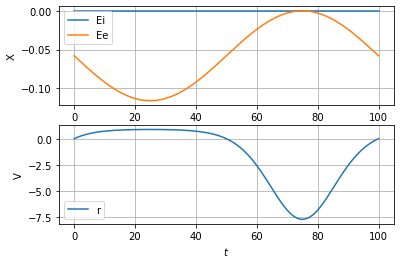In :
def PlotClamp():
t = dat['t']
phi_Ei = dat['phi'][:,s['species'].index('Ei')]
phi_Ee = dat['phi'][:,s['species'].index('Ee')]
x_Ii = dat['X'][:,s['species'].index('Ii')]
x_Ie = dat['X'][:,s['species'].index('Ie')]
v = dat['V'][:,s['reaction'].index('r')]
V_N = st.V_N()

dV = phi_Ei-phi_Ee

## BG
v_BG = (1/x_Ii)*(x_Ii - x_Ie*np.exp(-dV/V_N))

## GHK
v_GHK = 0.5*v_BG*(dV/V_N)/(1-np.exp(-dV/V_N))

## HH
v_HH = (np.exp(-V_Nernst))*(dV - V_Nernst)/V_N

plt.plot(dV*1000,v,label='Clamp',lw = 6)
plt.plot(dV*1000,v_BG,label='BG')
plt.plot(dV*1000,v_GHK,label='GHK')
plt.plot(dV*1000,v_HH,label='HH')
plt.vlines(1000*V_Nernst,min(v),max(v),linestyle='dashed')
plt.grid()
plt.legend()
plt.xlabel('$\Delta E$ mV')
plt.ylabel('$v/\kappa$')
##plt.savefig('Figs/clamp.pdf')

PlotClamp()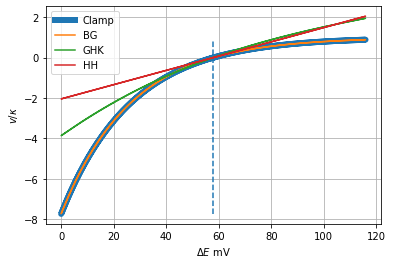# Gated ion channel¶

In :
## Ion Channel
sbg.model('IonChannel_abg.svg')
import IonChannel_abg
disp.SVG('IonChannel_abg.svg')

Out:
In :
## Stoichiometry
s = st.stoich(IonChannel_abg.model(),linear=['Ei','Ee'],quiet=quiet)
if Fix_conc:
chemostats = ['Ii','Ie','G']
else:
chemostats = ['G']
sc = st.statify(s,chemostats=chemostats)
print(s['species'])

['Ee', 'Ei', 'G', 'Ie', 'Ii']

In :
## Reactions
disp.Latex(st.sprintrl(s,chemformula=True,all=True))

Out:
\begin{align} \ch{Ei + G + Ii &<>[ r ] Ee + G + Ie } \end{align}
In :
## Flows
disp.Latex(st.sprintvl(s))

Out:
\begin{align} v_{r} &= K_{G} \kappa_{r} x_{G} \left(- K_{Ie} x_{Ie} e^{\frac{K_{Ee} x_{Ee}}{V_{N}}} + K_{Ii} x_{Ii} e^{\frac{K_{Ei} x_{Ei}}{V_{N}}}\right) \end{align}
In :
X_chemo = {'G':'0.1'}
dat = Simulate(s,sc,X_chemo=X_chemo)
#st.plot(s,dat)
st.plot(s,dat,plotPhi=True,species=['Ei','Ee'])

Na
dV = 57.4mV
dV_Theory = 57.9mV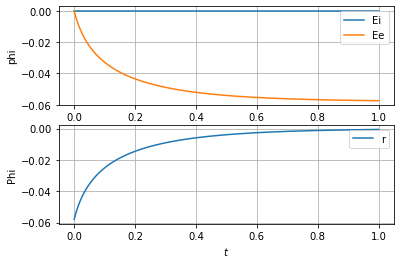# Interacting ion channels¶

Two instances of the ion channel module are combined; one corresponds to Na$^+$ and one to K$^+$. The species concentations are encapsulated in the individual modules, but the electrical capaciter are shared. This is a simplified version of the Hodgkin-Huxley model of the squid giant axon and the correponding Na$^+$ and K$^+$ concentrations are used.

The simulations use piecewise constant gating variables $G_{Na}$ and $G_{K}$: \begin{align} G_K &= \begin{cases} 10^{-6} & \text{for $0.3<t<0.35$}\\ 1 & \text{otherwise} \end{cases}\\ G_{Na} &= \begin{cases} 1 & \text{for $0.3<t<0.35$}\\ 4.3 \times 10^{-3} & \text{otherwise} \end{cases} \end{align}

The time course of the membrane potential $\Delta E$ can be explained as follows.

$t<0.3$: $\Delta E$ moves from the initial condition of zero to a resting potential of about $-65$mV. This corresponds to the value in Table 2.1 of Keener \& Sneyd; the resting potential depends not only on Nernst potentials of Na$^+$ and K$^+$ (which in turn depends on the concentrations) but also on the values of the gating potential.

$0.3<t<0.35$: $\Delta E$ undergoes a typical action potential as the Na$^+$ gate opens and moves toward the Nernst potential for Na$^+$ until the gate closes.

$t>0.35$: $\Delta E$ returns to the resting potential.

In this simple example the gating variables $G_{Na}$ and $G_{K}$ are independent variables, in reality, and in the HH model, the gating variables are modulated by the membrane potential $\Delta E$. This is discussed in a bond graph context by \citet{GawSieKam17}.

In :
## Ion Channels
sbg.model('IonChannels_abg.svg')
import IonChannels_abg
disp.SVG('IonChannels_abg.svg')

Creating subsystem: IonChannel:K
Creating subsystem: IonChannel:Na

Out:
In :
## Stoichiometry
s = st.stoich(IonChannels_abg.model(),linear=['Ei','Ee'],quiet=quiet)
if Fix_conc:
chemostats = ['Na_Ii','Na_Ie','Na_G', 'K_Ii','K_Ie','K_G']
else:
chemostats = ['Na_G','K_G']
sc = st.statify(s,chemostats=chemostats)
print(s['species'])

['Ee', 'Ei', 'K_G', 'K_Ie', 'K_Ii', 'Na_G', 'Na_Ie', 'Na_Ii']

In :
## Reactions
disp.Latex(st.sprintrl(s,chemformula=True,all=True))

Out:
\begin{align} \ch{Ei + K_G + K_Ii &<>[ K_r ] Ee + K_G + K_Ie }\\ \ch{Ei + Na_G + Na_Ii &<>[ Na_r ] Ee + Na_G + Na_Ie } \end{align}
In :
## Flows
disp.Latex(st.sprintvl(s))

Out:
\begin{align} v_{K r} &= K_{K G} \kappa_{K r} x_{K G} \left(- K_{K Ie} x_{K Ie} e^{\frac{K_{Ee} x_{Ee}}{V_{N}}} + K_{K Ii} x_{K Ii} e^{\frac{K_{Ei} x_{Ei}}{V_{N}}}\right)\\ v_{Na r} &= K_{Na G} \kappa_{Na r} x_{Na G} \left(- K_{Na Ie} x_{Na Ie} e^{\frac{K_{Ee} x_{Ee}}{V_{N}}} + K_{Na Ii} x_{Na Ii} e^{\frac{K_{Ei} x_{Ei}}{V_{N}}}\right) \end{align}
In :
t0_Na = 0.3
t1_Na = 0.35
t0_K = 0.35
t1_K = 1.0

G_K_0 = 1e-6
G_Na_0 = 4.3e-3

G_K = f'{G_K_0}+np.heaviside(t,1)-np.heaviside(t-{t0_Na},1)+np.heaviside(t-{t0_K},1)-np.heaviside(t-{t1_K},1)'
G_Na = f'{G_Na_0}+np.heaviside(t-{t0_Na},1)-np.heaviside(t-{t1_Na},1)'
X_chemo = {'Na_G':G_Na,'K_G':G_K}
dat = Simulate(s,sc,X_chemo=X_chemo,prefix=['Na_','K_'],T=0.6)
st.plot(s,dat,plotPhi=True,species=['Ei','Ee'])

Na
K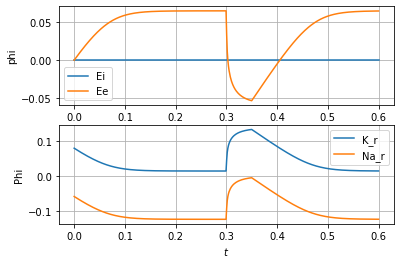In :
def PlotAction():
t = dat['t']
phi_Ei = dat['phi'][:,s['species'].index('Ei')]
phi_Ee = dat['phi'][:,s['species'].index('Ee')]
dE = phi_Ei-phi_Ee

print(f'Resting potential = {1000*dE[-1]:.2f} mV')

X_G_K = dat['X'][:,s['species'].index('K_G')]
X_G_Na = dat['X'][:,s['species'].index('Na_G')]

v_Na = dat['V'][:,s['reaction'].index('Na_r')]
v_K  = dat['V'][:,s['reaction'].index('K_r')]

conc_Na_e = K_Ie*dat['X'][:,s['species'].index('Na_Ie')]
conc_Na_i = K_Ii*dat['X'][:,s['species'].index('Na_Ii')]
conc_Na_e_0 = conc_Na_e
conc_Na_i_0 = conc_Na_i

conc_K_e = K_Ie*dat['X'][:,s['species'].index('K_Ie')]
conc_K_i = K_Ii*dat['X'][:,s['species'].index('K_Ii')]
conc_K_e_0 = conc_K_e
conc_K_i_0 = conc_K_i

plt.plot(t,1000*dE)
plt.grid()
plt.ylabel('$\Delta E$ mV')
plt.xlabel('$t$')
#plt.savefig('Figs/action.pdf')
plt.show()

plt.plot(t,v_Na,label='Na')
plt.plot(t,v_K,label='K')
plt.grid()
plt.legend()
plt.ylabel('$i$ mA')
plt.xlabel('$t$')
#plt.savefig('Figs/action_current.pdf')
plt.show()

plt.plot(t,100*(conc_Na_e-conc_Na_e_0)/conc_Na_e_0,label='Na_e')
plt.plot(t,100*(conc_Na_i-conc_Na_i_0)/conc_Na_i_0,label='Na_i')
plt.plot(t,100*(conc_K_e-conc_K_e_0)/conc_K_e_0,label='K_e')
plt.plot(t,100*(conc_K_i-conc_K_i_0)/conc_K_i_0,label='K_i')

plt.legend()
plt.grid()
plt.ylabel(r'$\Delta c (\%)$')
plt.xlabel('$t$')
##plt.savefig('Figs/action_conc.pdf')
plt.show()

plt.plot(t,X_G_K,label='G_K')
plt.plot(t,X_G_Na,label='G_Na')
plt.legend()
plt.grid()
plt.ylabel('Gating')
plt.xlabel('$t$')
##plt.savefig('Figs/action_gating.pdf')
plt.show()

PlotAction()

Resting potential = -64.90 mV